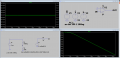# Using LTSpice to built a opamp with arbitrary behavior voltage source

#### aarontan0219

Joined Nov 16, 2019
22
I had built up a circuit using arbitrary behavior voltage source and set it up same as an opamp but just changed the input and output resistance as well as the Avo to the required one from the questions. It acts normally when doing DC analysis to find the offset voltage as well as act as an non inverting amplifier. But when I started trying to do AC analysis to find the unity gain bandwidth with open-loop no load condition, it dosent get the slope as I simulated with an opamp. The graph and circuit is the one using arbitrary behavior voltage source while for the right one is the one using the ordinary opamp.#### ericgibbs

Joined Jan 29, 2010
17,419
hi 219,
Look at page 17 of this PDF, Note Cc .! 2nd Stage
E

Edited.

#### Attachments

• 273.2 KB Views: 12

#### aarontan0219

Joined Nov 16, 2019
22

#### ericgibbs

Joined Jan 29, 2010
17,419

#### aarontan0219

Joined Nov 16, 2019
22
Hi Eric,
This is my .asc file.

#### Attachments

• 759 bytes Views: 6

#### crutschow

Joined Mar 14, 2008
32,043
You need the equivalent of the Cc compensation capacitor, which provides closed-loop stability in an op amp.
That's what generates the observed rolloff of the opamp model.

#### aarontan0219

Joined Nov 16, 2019
22
You need the equivalent of the Cc compensation capacitor, which provides closed-loop stability in an op amp.
That's what generates the observed rolloff of the opamp model.
Hi,
can you guide me through it?

#### ericgibbs

Joined Jan 29, 2010
17,419
hi,

How did you derive the func : satlimit and have you done an actual evaluation.

As Carl reminds you Cc, is very important.

E

#### aarontan0219

Joined Nov 16, 2019
22
hi,

How did you derive the func : satlimit and have you done an actual evaluation.

As Carl reminds you Cc, is very important.

E
Basically satlimit is the name of the function and Vcc is the value selected, in my case the Vcc for the opamp will be 15. Then The V(+) is the non inverting input and the 140k is my Avo.

#### ericgibbs

Joined Jan 29, 2010
17,419
hi,
All OPA's have an output impedance typically around 50R, and say a internal Cc of 100pF.

Try modelling that combination.
E

#### MrAl

Joined Jun 17, 2014
10,075
Hello,

Probably the simplest model of an op amp with a particular frequency response is not quite a voltage controlled voltage source but a voltage controlled current source along with an output resistor and capacitor in parallel. So it is almost the same as the VCVS but it has a current source output that works with the R and C that creates the roll off.
The resistor is made small (like 1 Ohm) and the capacitor is then chosen for the desired frequency response. Since there is a frequency response roll off, the internal gain (which is the gain factor of the VCCS) can not be infinite but must be something similar to a real life op amp. That means you get a tiny inaccuracy the same as you would with a real life op amp too.

The response when the non inverting terminal is grounded then looks like this:
Vout=-(vn*A*R)/(s*C*R+1)
where vn is the voltage applied to the inverting terminal.
With R=1 as noted, this reduces to:
Vout=-(vn*A)/(s*C+1)

and you can see this open loop response acts like a low pass filter and if you compute the amplitude and plot it with log log scales you get the response you had shown earlier.
Also notable and simple (when R=1) is the slew rate can be approximated as A in volts per second. So if you make A=100000 then the slew rate is 0.1 v/us. It's not exactly the same as a real life op amp but it works decently.

As note the resistor was made equal to 1 Ohm, so the impedance of the load also plays a part in the response if the R and C of the load is comparable to the R and C of the op amp model. This has to be accounted for if it is, but usually the output would be something like 1k which is 1000 times greater than the 1 Ohm model output resistance. If this really works out to a problem then you can use a unity gain output buffer with no roll off.